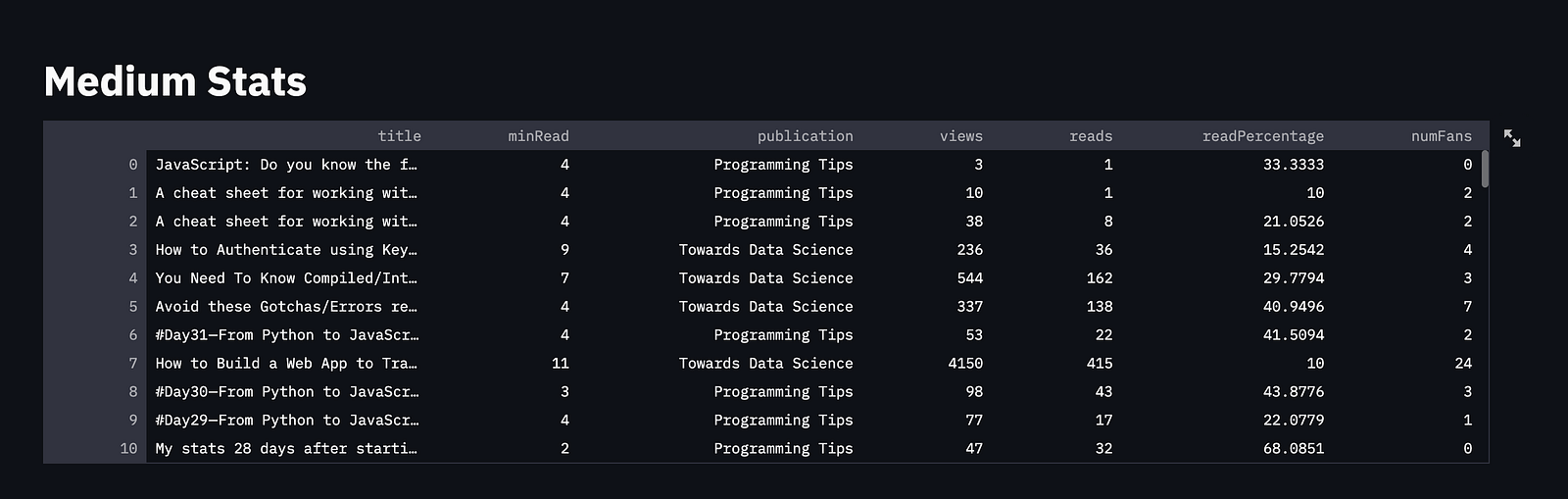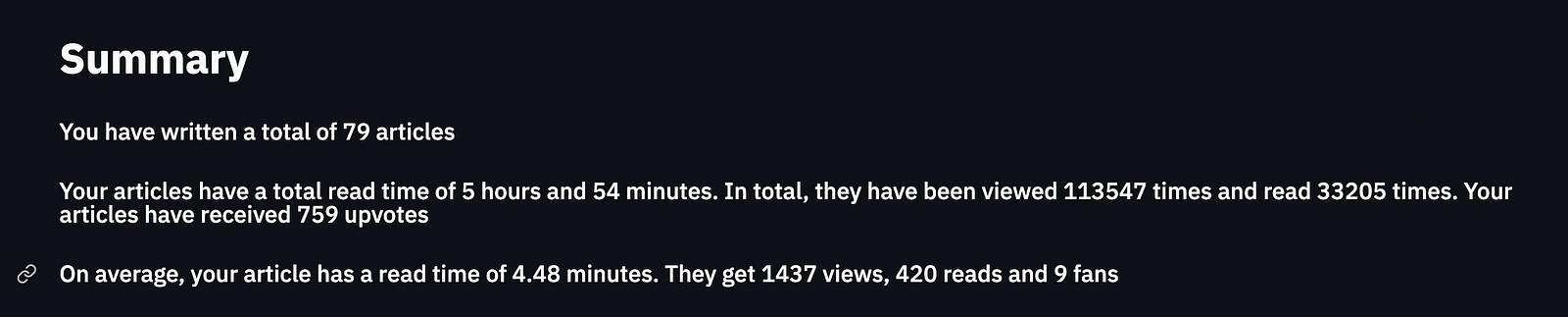# .css-4zleql{display:block;}Rahul Banerjee# How to get a JSON file of your Medium Stats and create a Dashboard using Streamlit

Rahul Banerjee
·Jun 13, 2021·

Subscribe to my newsletter and never miss my upcoming articles

### How to get a JSON file of your Medium Stats and create a Dashboard using Streamlit

We will be doing the following

• Getting Medium Stats in a JSON format
• Use Pandas and Plotting libraries to plot charts
• Display the charts on a UI created using Streamlit

You can find the repo here

### Getting Medium Stats in a JSON format

Unfortunately Medium doesn’t seem to have any easy-to-use API publicly available so we will have to use a slightly hacky solution. We will essentially be scraping the stats, we will have to do it using JavaScript since it will have to run in your browser’s console. Check this article to see how you can access your browser’s console.

You don’t need to know JavaScript, you can run the script as-is. However, if you want more metrics/stats about your articles, you will have to tinker around with the script.

We will store the following data

• Title
• Read time of Each Article
• Publication the article was published in
• Number of Views
• Number of Fans

The JSON file will basically be an array of objects having the above properties.

You can find the script here

Basically, we will have to go to https://medium.com/me/stats and scroll all the way to the bottom, i.e scroll till you see all your articles. Run the script in your console and it will download a JSON file with all your stats.

### Setting Up Environment

• Create a new folder to store your JSON data and the Python files

``mkdir medium-analysis``

• Create Virtual Environment

``python3 -m venv venv``

• Activate Virtual Environment

``source venv/Bin/activate``

• Create Python File

``touch main.py``

• Install Required Libraries

``pip3 install streamlit,pandas,seaborn,matplotlib``

• Import the Libraries

``````import pandas as pd
import matplotlib.pyplot as plt
import streamlit as st
import seaborn as sns``````

• Run the streamlit app to ensure everything is working fin

``streamlit run main.py``

### Displaying DataFrame of Medium StatsScreenshot by Author

Before we begin, we will set the default theme to dark mode and the layout to wide mode.

``````plt.style.use("dark_background")
st.set_page_config(layout = "wide")``````

We will use pandas’ read_json() function to convert the JSON file to a dataframe.

``df = pd.read_json("story.json")``

Now, we will display a title and the dataframe in the UI

``````st.title("Medium Stats")
st.dataframe(df)``````

### Summary SectionScreenshot by Author

Our Summary section will basically consist of the sum of the various features in our dataframe and the average.

We can use Pandas’ sum() function to find the sum of a column and use the standard len() function to find the number of rows (articles) in our dataframe.

``````minReadSum = df['minRead'].sum()
totalNumViews = df['views'].sum()
totalNumFans = df['numFans'].sum()
numArticles = len(df)``````

To calculate the average, simply divide the sum by the length.

We will use the subheader() function in Streamlit to display the text.

``````st.subheader(f'You have written a total of {numArticles} articles')

### Top 5 By Column Name

Since we will be doing it for multiple columns, we will write a function that will accept the column name as a parameter and return a figure.

``````def plot_top_5(df, colName):
top5 = df.sort_values(by=[colName],ascending = False)[:5]
fig,ax = plt.subplots(figsize = (15,15)
ax = sns.barplot(x = colName, y = "title", data = top5)
return fig``````

The column is sorted the first 5 values are plotted on a bar graph

We will streamlit’s beta_columns() function to split the UI into two columns. Each column will contain one of the graphs

``````st.title("Your Top 5")
p1,p2 = st.beta_columns(2)
for idx,colName in enumerate(colNames):
curr = p1 if idx%2==0 else p2
curr.pyplot(plot_top_5(df, colName))``````

### Publication Distribution

Before we use the publications data, we will need to clean it up a bit. For the stories which were not published in any publication, you will notice that the publication is “View Story”. We will change this to “No Publication.

``df['publication'] = df.apply( lambda row : row['publication'] if row['publication'] != 'View story' else "No Publication" , axis = 1)``

The value_counts() function returns the occurrence of each type of publication. This can be plotted as a pie chart.

``````Publicationscount = df['publication'].value_counts()
fig = plt.figure(figsize=(20,20))
plt.pie(Publicationscount, labels = Publicationscount.index,autopct='%1.0f%%')
p1.pyplot(fig)``````

This chart will be plotted in the first column we created earlier.

Now will plot a chart to showcase the number of articles in various read-time intervales, i.e Number of articles with read time of 1 -3 mins, Number of articles with read time of 4–7 mins, etc

First, we will need to classify the articles based on their read time. Let's write a function to do so.

``````def categorizeArticle(readTime):
return '1-3 mins'
elif 4 <= readTime <= 6:
return '4-6 mins'
return '7-9 mins'
else:
return '9+ mins'``````

The above function will be called on each row of the dataframe and the read time of the article will be passed in as an argument.

``df['articleCategory'] = df.apply(lambda row: categorizeArticle(row['minRead']) , axis =1)``

This will create a new categorical column.

Finally, we will plot it as a countplot

``````p2.title("Article Read Time")
fig,ax = plt.subplots(figsize=(25,25))
ax = sns.countplot(data=df,x='articleCategory',order=['1-3 mins','4-6 mins','7-9 mins','9+ mins'])
p2.pyplot(fig)``````

This will be plotted in the second column

### Conclusion

We can further build on top of this

• Plot Co-relation graphs between the features
• Update the Script to get the date the article was published and plot distribution of articles by months
• Update the Script to open the stats of the article and scrape the earnings, avg read time and member read time.